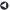### Statistical Services OfferedBack to List of Services

#### Analysis of Variance (ANOVA)

Analysis of variance (ANOVA) is a statistical technique used to compare the means of two or more groups. For ANOVA, the dependent (or response or outcome) variable must be continuous, and the independent (or predictor or explanatory) variable(s) must be discrete (or categorical). An example would be a study of the effect on blood pressure (a continuous variable) of medication type (a categorical variable); a third of the subjects are given the existing drug, a third are given a new drug, and a third are given a placebo. The goal of the study would be to determine if there are significant differences in the mean value of blood pressure between the three groups (or treatments). In One-Way ANOVA there is one categorical predictor variable, in Two-Way ANOVA there are two categorical predictor variables, and in N-Way ANOVA there are n categorical predictor variables.

Total variation in the data is partitioned into within-group variation and between-group variation, with the independent variable(s) explaining the between-group variation. The sums of their squared differences ("sum of squares") are used to develop an overall F-test for testing the ANOVA null hypothesis that all group means are equal. A statistically significant overall F-test enables you to reject the null hypothesis, but at this point all you know is that there is at least one group mean different from one other group mean. To find out which group means differ, you perform multiple comparison tests.

In a completely randomized design, treatments are randomly assigned to experimental units. However, this design does not isolate any of the variability due to extraneous causes. In a randomized block (RCB) design, treatments are randomly assigned within blocks. Blocks are groups of experimental units selected in such a way that experimental units within blocks are as homogeneous as possible. Blocks are designed to isolate variability due to extraneous causes. Both designs must take into account whether the data is balanced (in balanced data, all treatments have an equal sample size).

One of the assumptions for ANOVA is normally distributed error terms for each treatment. Although ANOVA is robust against minor departures from its normality assumption, extreme departures from normality can impair its sensitivity to differences between means. Therefore, when the data is very skewed or when there are extreme outliers, nonparametric methods may be more suitable. In addition, when the data is ordinal rather than interval, nonparametric methods should be used. (In nonparametric analysis, the rank of each data point is used instead of the raw data.) Even when the normality assumption is true, nonparametric tests perform almost as well as parametric tests.